• 此演示用于推荐系统改进矩阵分解 (MF) 公式% 遵循带有用户和项目元数据盲压缩感知 (BCS) 框架% 优化问题% 最小化_(U,V, A, C) ||YM(UV)||_F + lambda_u||U||_F + lambda_v||V||_1 + mu_u||W-UC||_F + mu_v|| Q-...matlab
• 除了它们描述诸如$$V \ rightarrow q \ bar {q}'$$ V→qq¯'之类衰减相关性之外，$$V = Z，W$$ V = Z，W，$$H \ rightarrow b \ bar {b}$$ H→bb和$$H \ rightarrow gg$$ H→gg，这些结果为计算嵌套...
• %d旋转角度x驱动位移关系 cosx =@(D) ((L2.^2-D.^2).^(0.5))./L2; D=0:800; G=0; I=3; for i=1:1:3 Lp=[1,2,3,4,5,6,7]; %积分上下限表达式 p=[3,2,1]; %d 定义多项式1...matlab 有问必答
• 何凯明大神代表作之一 论文地址：Guided Image Filtering 导向滤波一般表达方式 qi=∑jWij(I)pj ...假设在局部范围内，输出图导向图关系可以用一个线性模型表示： qi=akIi+bk,∀i∈ωk q_...
何凯明大神的代表作之一
论文地址：Guided Image Filtering
导向滤波的一般表达方式
$q_{i}=\sum_{j} W_{i j}(I) p_{j}$
其中$q$表示输出，$p$表示输入，$I$表示导向图。
先验假设
假设在局部范围内，输出图与导向图的关系可以用一个线性模型表示：
$q_{i}=a_{k} I_{i}+b_{k}, \forall i \in \omega_{k}$
另外输出图是由输入图减去噪声（需要被滤掉的部分）得到
$q_{i}=p_{i}-n_{i}$
能量函数和求解
我们需要做的就是最小化能量函数
$E\left(a_{k}, b_{k}\right)=\sum_{i \in \omega_{k}}\left(\left(a_{k} I_{i}+b_{k}-p_{i}\right)^{2}+\epsilon a_{k}^{2}\right)$
其中$\epsilon$为正则项，防止系数$a_k$过大。
对$a_k$和$b_k$求导：
$\frac{\partial E}{\partial a_{k}}=2 \sum_{i}^{N}\left(\left(-p_{i}+a_{k} I_{i}+b_{k}\right) I_{i}+\epsilon a_{k}\right)$
$\frac{\partial E}{\partial b_{k}}=-2 \sum_{i}^{N}\left(p_{i}-a_{k} I_{i}-b_{k}\right)$
先求取$b_k$，令偏导数为零：
\begin{aligned} &0= \sum_{i}^{N}\left(p_{i}-a_{k} I_{i}-b_{k}\right)\\ &\Rightarrow N b_{k}=\sum_{i}^{N}\left(p_{i}-a_{k} I_{i}\right)\\ &\Rightarrow b_{k}=\frac{1}{N} \sum_{i}^{N}\left(p_{i}-a_{k} I_{i}\right)\\ &\Rightarrow b_{k}=\frac{1}{N} \sum_{i}^{N} p_{i}-a_{k} \frac{1}{N} \sum_{i}^{N} I_{i} \\ &\Rightarrow b_{k}= \bar{p_{k}}-a_{k} \mu_{k} \end{aligned}
其中$\bar{p_k}$为输入图窗口内的平均值，$\mu_k$为导向图在窗口内的平均值。
同理，再来求$a_k$
\begin{aligned} &0= \sum_{i}^{N}\left(\left(-p_{i}+a_{k} I_{i}+b_{k}\right) I_{i}+\epsilon a_{k}\right)\\ &\Rightarrow 0=\sum-p_{i} I_{i}+\sum a_{k} I_{i}^{2}+\sum b_{k} I_{i}+\sum \varepsilon a_{k}\\ &\Rightarrow \sum p_{i} I_{i}-\sum b_{k} I_{i}=a_{k} \sum\left(I_{i}^{2}+\epsilon\right)\\ &\Rightarrow \sum p_{i} I_{i}-\sum\left(p_{k}-a_{k} \mu_{k}\right) I_{i}=a_{k} \sum\left(I_{i}^{2}+\epsilon\right)\\ &\Rightarrow a_{k}=\frac{\sum\left(p_{i} I_{i}-p_{k} I_{i}\right)}{\sum\left(I_{i}^{2}-\mu_{k} I_{i}+\epsilon\right)}\\ &\Rightarrow a_{k}=\frac{\sum\left(p_{i} I_{i}\right)-\frac{1}{N} \sum p_{i} \sum I_{i}}{\sum I_{i}^{2}-\frac{1}{N} \sum I_{i} \sum I_{i}+\epsilon}=\frac{\frac{1}{N} \sum\left(p_{i} I_{i}\right)-\frac{1}{N} \sum p_{i} \frac{1}{N} \sum I_{i}}{\frac{1}{N} \sum I_{i}^{2}-\frac{1}{N} \sum I_{i} \frac{1}{N} \sum I_{i}+\epsilon} \end{aligned}
根据方差和协方差公式
\begin{aligned} &\operatorname{var}(X)=\frac{\sum_{i=1}^{n}\left(X_{i}-\bar{X}\right)^2}{n-1}\\ &\operatorname{Cov}(X, Y)=E[(X-E[X])(Y-E[Y])]\\ &\operatorname{Cov}(X, Y)=E[X Y]-E[X] E[Y] \end{aligned}
可以得到
$a_{k}=\frac{\operatorname{Cov}(p, I)}{\operatorname{Var}(I)+N \epsilon}=\frac{\operatorname{Cov}(p, I)}{\sigma_{k}^{2}+\epsilon}$
当导向图与输入图相同时，导向滤波就变成了以输入图自身为导向的保边滤波。上面表达式就会变成
\begin{aligned} a_{k} &=\frac{\sigma_{k}^{2}}{\sigma_{k}^{2}+\varepsilon} \\ b_{k} &=\left(1-a_{k}\right) \mu_{k} \end{aligned}

当遇到边缘区域，方差较大，此时$\sigma_{k}^2>>\epsilon$，有$q=p$
反之，当方差较小，$\sigma_{k}^2<<\epsilon$，输出均值$q=\mu_k$

其实更仔细思考一下，这里对“边缘”和“平坦”区域的定义实际上是取决于$\epsilon$的取值，当区域方差远大于$\epsilon$时，则“定义为”这次滤波中的边缘区域，在滤波时会被保留，反之则是平坦区域。
思考
图像滤波与线性回归的关系
线性回归：有一些离散点$x_i$，需要拟合一条直线$y_i$使得$\epsilon=\sum_{i}(y_i-(ax_i+b))^2$最小。
图像去噪：有一些含有噪声的像素$p_i$，需要拟合一个线性函数找到没有噪声的图像$q_i$，使得$\epsilon=\sum_{i}(p_i-q_i)^2$最小。
扩展
简化计算
可以看到按照滤波公式，对于输出的每一个像素，都需要计算输入图和导向图在窗口内的均值，以此得到$a_k$和$b_k$。但是这其中有很多重复计算，此时可以通过计算经过所有覆盖当前像素的窗口的平均$a_k$和$b_k$来计算单个像素的输出
$q_{i}=\bar{a}_{i} I_{i}+\bar{b}_{i}$
经过这个改变之后可能无法满足最初的$\nabla q = a\nabla I$，但是仍然可以保证在强边缘附近导向图的信息是可以被保留下来的$\nabla q \approx \bar{a} \nabla I$。
滤波核计算
根据最开始的一般表达式，可以知道输出$q_i$是线性依赖于输入$p_i$，所以要求取滤波核只需要使用$q_i$对$p_i$求导就可以。将上面表达式中的$b_k$替换掉，可以得到
$q_{i}=\frac{1}{|\omega|} \sum_{k \in \omega_{i}}\left(a_{k}\left(I_{i}-\mu_{k}\right)+\bar{p}_{k}\right)$
$\frac{\partial q_{i}}{\partial p_{j}}=\frac{1}{|\omega|} \sum_{k \in \omega_{i}}\left(\frac{\partial a_{k}}{\partial p_{j}}\left(I_{i}-\mu_{k}\right)+\frac{\partial \bar{p}_{k}}{\partial p_{j}}\right)$
其中
$\frac{\partial \bar{p}_{k}}{\partial p_{j}}=\frac{1}{|\omega|} \delta_{j \in \omega_{k}}=\frac{1}{|\omega|} \delta_{k \in \omega_{j}}$
是一个狄拉克函数，在窗口内部为1，其余地方为0。
在根据$a_k$的表达式对$p_j$求导
\begin{aligned} \frac{\partial a_{k}}{\partial p_{j}} &=\frac{1}{\sigma_{k}^{2}+\epsilon}\left(\frac{1}{|\omega|} \sum_{i \in \omega_{k}} \frac{\partial p_{i}}{\partial p_{j}} I_{i}-\frac{\partial \bar{p}_{k}}{\partial p_{j}} \mu_{k}\right) \\ &=\frac{1}{\sigma_{k}^{2}+\epsilon}\left(\frac{1}{|\omega|} I_{j}-\frac{1}{|\omega|} \mu_{k}\right) \delta_{k \in \omega_{j}} \end{aligned}
代回原式就得到了滤波核的表达式
$\frac{\partial q_{i}}{\partial p_{j}}=\frac{1}{|\omega|^{2}} \sum_{k \in \omega_{i}, k \in \omega_{j}}\left(1+\frac{\left(I_{i}-\mu_{k}\right)\left(I_{j}-\mu_{k}\right)}{\sigma_{k}^{2}+\epsilon}\right)$
根据滤波核表达式，当像素$i$和$j$都在窗口内部，但处于一个边缘的同侧时（颜色相近），括号内后半部分的计算结果为正，融合权重很高；反之如果处于边缘的异侧，括号内后半部分结果为负，融合权重就会小很多。
高斯导向滤波
由于导向滤波算法采用的是均值滤波，在纹理不那么强或是均匀纹理时，导向滤波会退化成两个均值滤波的串联，多次均值滤波的串联虽然可以近似高斯滤波，但是仅两次均值滤波还是会造成权重在x和y方向附近比其他方向稍大，如图
这是可以在能量函数前面加一个高斯核，让权重可以更加均匀分布
$E\left(a_{k}, b_{k}\right)=\sum_{i \in \omega_{k}} w_{i k}\left(\left(a_{k} I_{i}+b_{k}-p_{i}\right)^{2}+\epsilon a_{k}^{2}\right)$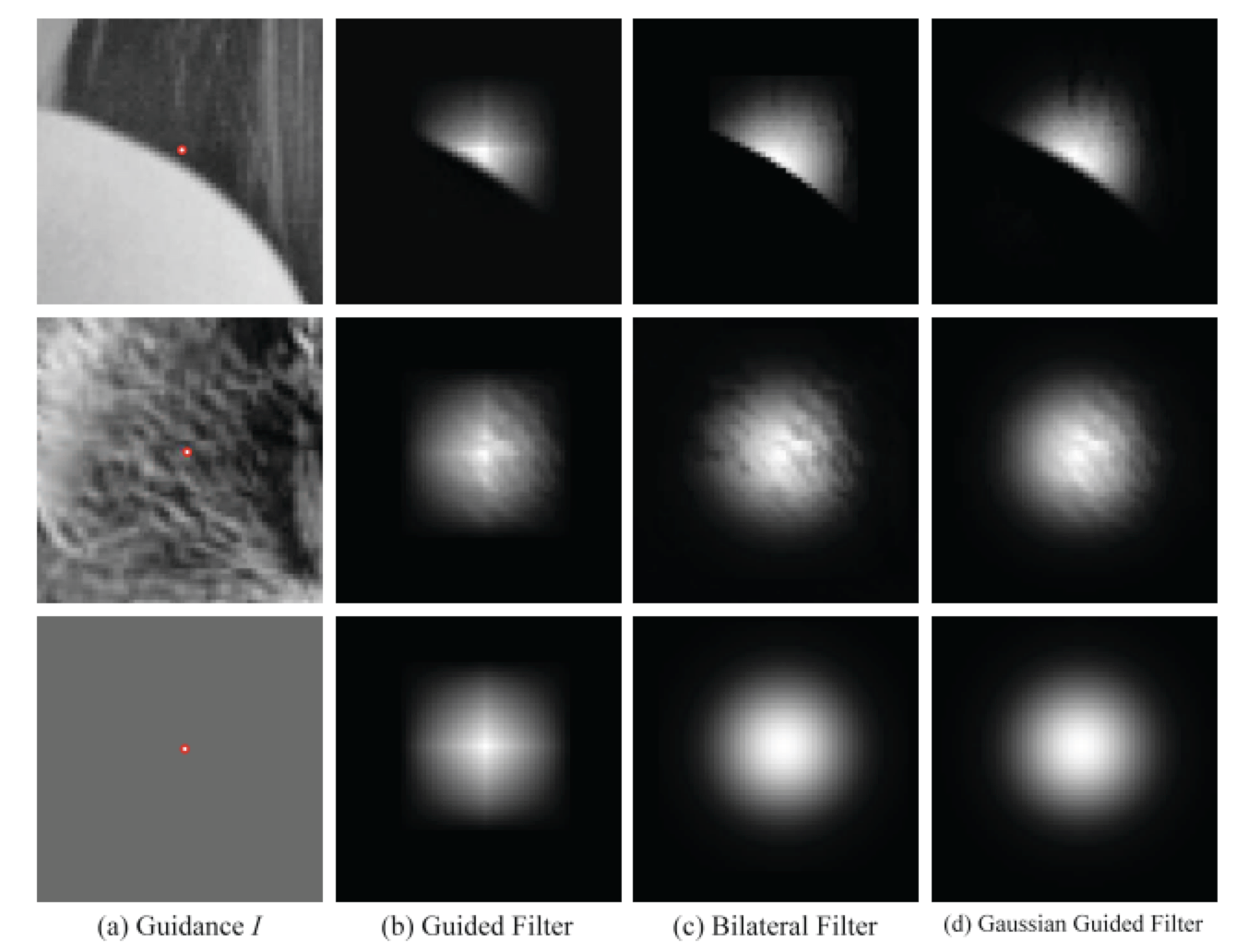Gradient-preserving
双边滤波会存在一个问题，主要体现在对边缘做滤波的时候，由于边缘像素本身和周围像素在数值上差距较大，会导致由数值差距判断的权重都很小，这时候融合权重就主要取决于高斯核，就可能融合出很多奇怪的效果，例如原本平滑过渡的边缘，会变得更加锐利，或者边缘过渡会变得不平滑，甚至出现梯度反向。经作者实验导向滤波是可以减轻甚至消除这种问题。


展开全文计算机视觉 算法
• 电容中存储电荷Q等于电容两端电压V与容量C乘积。...电容所能存储电场能量W与电容两端电压V及电容容量C有关。 假如某电容两端电压为9V,容量为470uF,则它所存储能量为0.019焦耳。 ...
电容中存储的电荷Q等于电容两端电压V与容量C的乘积。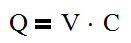流经电容的电流与电压并不是简单的线性关系。而是用下面的公式来计算：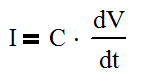电流I等于电容容量C乘以电容两端电压的变化率，式中，dV/dt表示电容两端电压的变化率，C是电容的容量。

电容所能存储的电场能量W与电容两端的电压V及电容的容量C有关。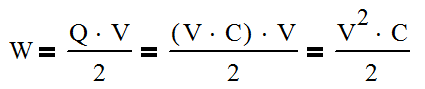假如某电容两端电压为9V,容量为470uF,则它所存储的能量为0.019焦耳。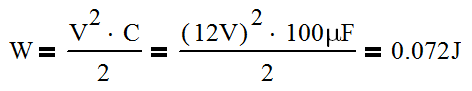展开全文• W玻色子对衰变在2ℓ2ν和ℓν2q最终状态下重建（ℓ= e或μ）。 考虑了信号胶子融合和矢量玻色子融合产生。 还考虑了信号背景之间干扰效应。 观察到数据标准模型（SM）预期一致。 横截面和支化分数乘积...
• 实现了重复频率高达100 kHz,紧凑全固态激光二极管抽运声光调Q 473 nm腔内倍频蓝光激光器。使用5 mm长Nd∶YAG...此公式与以前结果不同,认为有效储能时间不等于上能级寿命。通过实验结果分析验证了这个结论。
• 实现了重复频率高达105 kHz紧凑全固态声光（A-O)调Q 532 nm腔内倍频...给出了平均功率重复频率关系一般公式,并提出即使是在四能级系统中,有效储能时间也并不等于上能级寿命,理论计算结果实验结果吻合得很好。
• 毫安时mAh瓦时Wh计算 毫安时（mAh）---（q） 瓦时（Wh）-------（W） 电压（V）----------（U） 公式W=qU 举例：8Wh/3.7V ≈ 2.162Ah=2162mAh 单位换算 1Wh=0.001kWh W/U=q 1Wh/1V=1Ah=1000mAh...
毫安时mAh与瓦时Wh的计算
毫安时（mAh）---（q）
瓦时（Wh）-------（W）
电压（V）----------（U）
公式：W=qU
举例：8Wh/3.7V ≈ 2.162Ah=2162mAh
单位换算
1Wh=0.001kWh
W/U=q
1Wh/1V=1Ah=1000mAh
1000mAh*1V=1Wh

展开全文• 1 四元数 1.1 理论基础 在我们能够完全理解四元数之前，我们必须先知道四元数是怎么来。四元数根源其实是复数。 四元数概念是由爱尔兰数学家Sir William Rowan Hamilton发明, ... q=w+xi+yj+zkq=w+xi+...
1 四元数

1.1 理论基础

在我们能够完全理解四元数之前，我们必须先知道四元数是怎么来的。四元数的根源其实是复数。

四元数的概念是由爱尔兰数学家Sir William Rowan Hamilton发明的, 公式是：

i2=j2=k2=ijk=−1${i}^{2}={j}^{2}={k}^{2}=ijk=-1$$i^2 = j^2 = k^2 = ijk = -1$
一般表达式：
q=w+xi+yj+zk$q=w+xi+yj+zk$$q = w + xi + yj + zk$

性质：
|q|2=w2+x2+y2+z2=1$|q{|}^{2}={w}^{2}+{x}^{2}+{y}^{2}+{z}^{2}=1$$|q|^2 = w^2 + x^2 + y^2 + z^2 = 1$

其中：i，j，k都是复数。并且通过公式：
i2=j2=k2=ijk${i}^{2}={j}^{2}={k}^{2}=ijk$$i^2 = j^2 = k^2 = ijk$
可以推出：
ij=k$ij=k$$ij = k$
jk=i$jk=i$$jk = i$
ki=j$ki=j$$ki = j$
通过公式：
ijk=−1$ijk=-1$$ijk = -1$
i−1=−i${i}^{-1}=-i$$i^{-1}= -i$
j−1=−j${j}^{-1}=-j$$j^{-1}= -j$
k−1=−k${k}^{-1}=-k$$k^{-1}= -k$

可以推出：
ji=−k$ji=-k$$ji = -k$
kj=−i$kj=-i$$kj = -i$
ik=−j$ik=-j$$ik = -j$

你可能已经注意到了，i、j、k之间的关系非常像笛卡尔坐标系下 单位向量的叉积规则：
x⃗ ×y⃗ =z⃗  ⟶ y⃗ ×x⃗ =−z⃗ ${ \vec x \times \vec y= \vec z} { \space \longrightarrow \space} { \vec y \times \vec x=− \vec z}$
y⃗ ×z⃗ =x⃗  ⟶ z⃗ ×y⃗ =−x⃗ ${ \vec y \times \vec z= \vec x} { \space \longrightarrow \space} { \vec z \times \vec y=− \vec x}$
z⃗ ×x⃗ =y⃗  ⟶ x⃗ ×z⃗ =−y⃗ ${ \vec z \times \vec x= \vec y} { \space \longrightarrow \space} { \vec x \times \vec z=− \vec y}$

Hamilton自己也发现i、j、k虚数，可以被用来表达3个笛卡尔坐标系的单位向量i、j、k，并且仍然保持有虚数的性质，也即： i2=j2=k2=−1${i}^{2}={j}^{2}={k}^{2}=-1$$i^2=j^2=k^2=−1$。

1.1.1 三维空间下：

向量差乘即向量积可以被定义为：
|a⃗ ×b⃗ |=|a⃗ |⋅|b⃗ |⋅sinθ$|\stackrel{\to }{a}×\stackrel{\to }{b}|=|\stackrel{\to }{a}|\cdot |\stackrel{\to }{b}|\cdot \mathrm{sin}\theta$$| \vec a \times \vec b |= | \vec a | \cdot | \vec b | \cdot \sin \theta$

叉乘(向量的外积)是物理里面常常用到的概念, 它是由两个向量得到一个新的向量的运算。一般我们都是从几何意义下手: 向量 a⃗ $\stackrel{\to }{a}$$\vec a$ 和向量 b⃗ $\stackrel{\to }{b}$$\vec b$ 叉乘，得到的是一个垂直于 a⃗ $\stackrel{\to }{a}$$\vec a$ 和 b⃗ $\stackrel{\to }{b}$$\vec b$ 的向量，c⃗ =a⃗ ×b⃗ $\stackrel{\to }{c}=\stackrel{\to }{a}×\stackrel{\to }{b}$$\vec c = \vec a \times \vec b$ 它的方向由右手螺旋法则确定, 它的长度是 a⃗ $\stackrel{\to }{a}$$\vec a$ 和 b⃗ $\stackrel{\to }{b}$$\vec b$ 组成的一个平行四边形的面积。

如下图所示：

![这里写图片描述](https://img-blog.csdn.net/20180427161117323?watermark/2/text/aHR0cHM6Ly9ibG9nLmNzZG4ubmV0L0Rpbm5lckhvd2U=/font/5a6L5L2T/fontsize/400/fill/I0JBQkFCMA==/dissolve/70)

叉乘满足的基本的性质如下:

- a⃗ ×a⃗ =0⃗ $\stackrel{\to }{a}×\stackrel{\to }{a}=\stackrel{\to }{0}$$\vec a \times \vec a = \vec 0$： 因为夹角是0, 所以平行四边形面积也是0, 即叉积长度为0；
- a⃗ ×b⃗ =−(b⃗ ×a⃗ )$\stackrel{\to }{a}×\stackrel{\to }{b}=-\left(\stackrel{\to }{b}×\stackrel{\to }{a}\right)$$\vec a \times \vec b = -(\vec b \times \vec a)$：等式两边的叉积等大反向, 模长因为平行四边形不变而相同, 方向因为右手法则旋转方向相反而相反；
- (λa⃗ )×b⃗ =λ(a⃗ ×b⃗ )$\left(\lambda \stackrel{\to }{a}\right)×\stackrel{\to }{b}=\lambda \left(\stackrel{\to }{a}×\stackrel{\to }{b}\right)$$(\lambda \vec a)\times \vec b = \lambda (\vec a \times \vec b)$：这点比较好想, 因为:
4. 正数 λ$\lambda$$\lambda$ 数量乘不会影响 a⃗ $\stackrel{\to }{a}$$\vec a$ 的方向, 所以左右的叉积方向一样; 负数 λ$\lambda$$\lambda$ 使得 a⃗ $\stackrel{\to }{a}$$\vec a$ 反向了, 但也使得左右叉积方向相反.
5. 对 a⃗ $\stackrel{\to }{a}$$\vec a$ 进行缩放, 平行四边形面积也同等缩放.

- (a⃗ +b⃗ )×c⃗ =a⃗ ×c⃗ +b⃗ ×c⃗ $\left(\stackrel{\to }{a}+\stackrel{\to }{b}\right)×\stackrel{\to }{c}=\stackrel{\to }{a}×\stackrel{\to }{c}+\stackrel{\to }{b}×\stackrel{\to }{c}$$(\vec a + \vec b)\times \vec c = \vec a \times \vec c + \vec b \times \vec c$：这最难想象的了放弃

1.1.2 四维空间下：

同理将i、j、k虚数用来表达3个笛卡尔坐标系单位向量X、Y、Z如下图所示：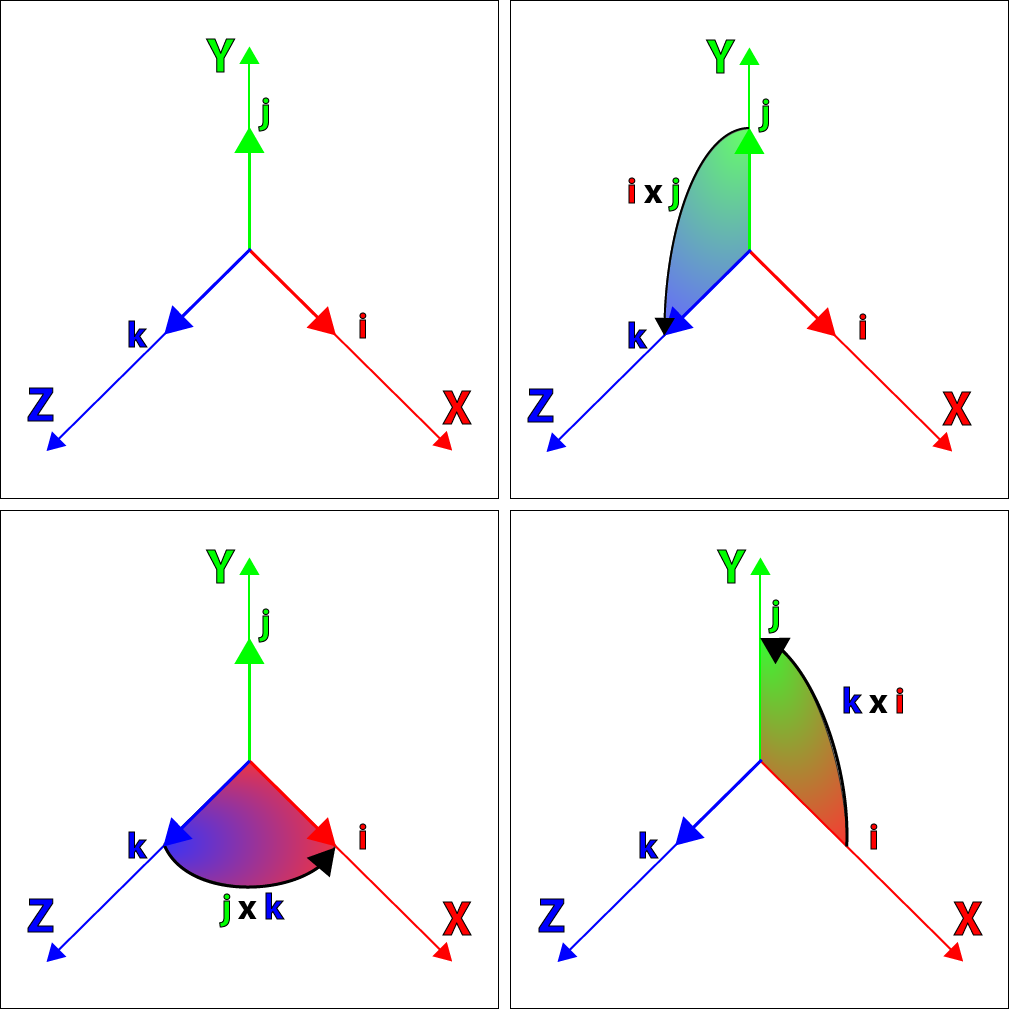可以得到：

i⃗ ×j⃗ =k⃗  ⟶ j⃗ ×i⃗ =−k⃗ ${ \vec i \times \vec j= \vec k} { \space \longrightarrow \space} { \vec j \times \vec i=− \vec k}$
j⃗ ×k⃗ =i⃗  ⟶ k⃗ ×j⃗ =−i⃗ ${ \vec j \times \vec k= \vec i} { \space \longrightarrow \space} { \vec k \times \vec j=− \vec i}$
k⃗ ×i⃗ =j⃗  ⟶ i⃗ ×k⃗ =−j⃗ ${ \vec k \times \vec i= \vec j} { \space \longrightarrow \space} { \vec i \times \vec k=− \vec j}$

至此，可以将四元素看作空间中任意一个向量了，为了简便，使用有序对的形式表示一个四元素：
q=w+xi+yj+zk ⟶ [w,v⃗ ]${q = w + xi + yj + zk} { \space \longrightarrow \space} {[w, \vec v]}$

其中向量 v⃗ $\stackrel{\to }{v}$$\vec v$ 有 xi+yj+zk$xi+yj+zk$$xi + yj + zk$ ，x ，y，z轴3个方向的分量。

1.1.3 四元数加减：

和复数类似，四元数也可以被加减，假设有两个向量：

qa=[wa,a⃗ ]${q}_{a}=\left[{w}_{a},\stackrel{\to }{a}\right]$$q_a = [w_a, \vec a]$
qb=[wb,b⃗ ]${q}_{b}=\left[{w}_{b},\stackrel{\to }{b}\right]$$q_b = [w_b, \vec b]$

于是有

qa+qb=[wa+wb,a⃗ +b⃗ ]${q}_{a}+{q}_{b}=\left[{w}_{a}+{w}_{b},\stackrel{\to }{a}+\stackrel{\to }{b}\right]$$q_a + q_b = [w_a + w_b, \vec a + \vec b]$
qa−qb=[wa−wb,a⃗ −b⃗ ]${q}_{a}-{q}_{b}=\left[{w}_{a}-{w}_{b},\stackrel{\to }{a}-\stackrel{\to }{b}\right]$$q_a - q_b = [w_a - w_b, \vec a - \vec b]$

1.1.4 四元数的积：

qaqb=[wa,a⃗ ][wb,b⃗ ]${q}_{a}{q}_{b}=\left[{w}_{a},\stackrel{\to }{a}\right]\left[{w}_{b},\stackrel{\to }{b}\right]$$q_aq_b = [w_a, \vec a][w_b, \vec b]$
=(wa+xai+yaj+zak)(wb+xbi+ybj+zbk)$=\left({w}_{a}+{x}_{a}i+{y}_{a}j+{z}_{a}k\right)\left({w}_{b}+{x}_{b}i+{y}_{b}j+{z}_{b}k\right)$$= (w_a + x_ai + y_aj + z_ak)(w_b + x_bi + y_bj + z_bk)$
=(wawb−xaxb−yayb−zazb)$=\left({w}_{a}{w}_{b}-{x}_{a}{x}_{b}-{y}_{a}{y}_{b}-{z}_{a}{z}_{b}\right)$$= (w_aw_b - x_ax_b - y_ay_b - z_az_b)$
+(waxb+wbxa+yazb+ybza)i$+\left({w}_{a}{x}_{b}+{w}_{b}{x}_{a}+{y}_{a}{z}_{b}+{y}_{b}{z}_{a}\right)i$$+ (w_ax_b + w_bx_a + y_az_b + y_bz_a)i$
+(wayb+wbya+zaxb+zbxa)j$+\left({w}_{a}{y}_{b}+{w}_{b}{y}_{a}+{z}_{a}{x}_{b}+{z}_{b}{x}_{a}\right)j$$+ (w_ay_b + w_by_a + z_ax_b + z_bx_a)j$
+(wazb+wbza+xayb+xbya)k$+\left({w}_{a}{z}_{b}+{w}_{b}{z}_{a}+{x}_{a}{y}_{b}+{x}_{b}{y}_{a}\right)k$$+ (w_az_b+ w_bz_a + x_ay_b + x_by_a)k$

2. 欧拉角

首先介绍roll，pitch，yaw的概念：

2.1 Roll:横滚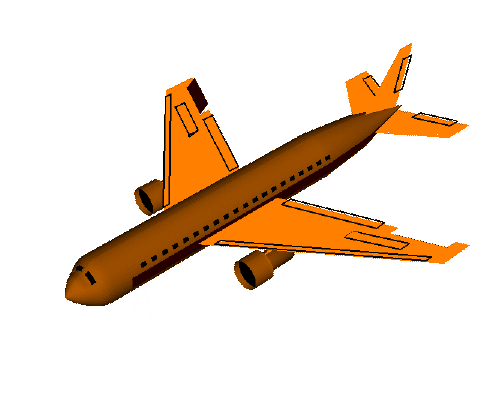2.2 Pitch: 俯仰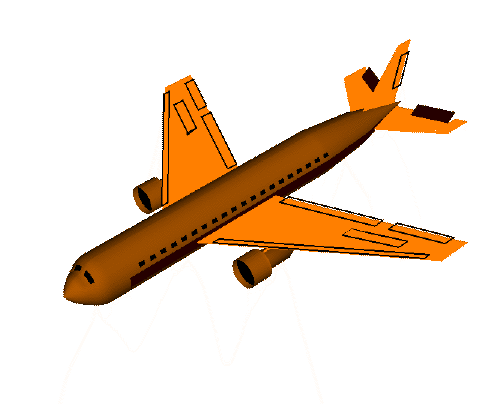2.3 Yaw: 偏航（航向）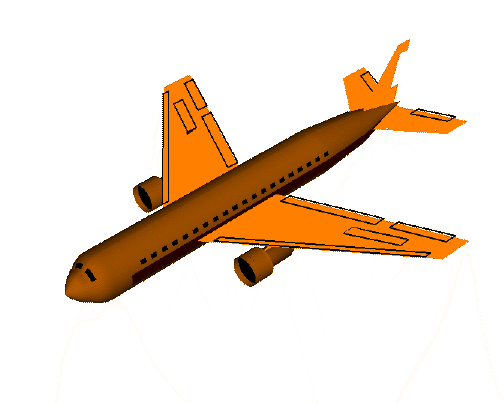在无人车或者机器上主要考虑的是Yaw偏航/航向角，因此这里不全部公式偏向于对yaw角的转换计算。

3. 四元数转欧拉角

假设有一个四元数的向量：Q(x,y,z,w)$Q\left(x,y,z,w\right)$$Q(x, y, z, w)$，绕轴A（ax，ay，az）$A（{a}_{x}，{a}_{y}，{a}_{z}）$$A（a_x，a_y，a_z）$旋转一个固定角度 α$\alpha$$\alpha$ , 将该动作分解为绕x，y，z（即ax，ay，az${a}_{x}，{a}_{y}，{a}_{z}$$a_x，a_y，a_z$）轴旋转角度roll，yaw，pitch，有以下公式：

|q|2=w2+x2+y2+z2=1$|q{|}^{2}={w}^{2}+{x}^{2}+{y}^{2}+{z}^{2}=1$$|q|^2 = w^2 + x^2 + y^2 + z^2 = 1$

⎡⎣⎢⎢rollpithyaw⎤⎦⎥⎥=⎡⎣⎢⎢ϕθψ⎤⎦⎥⎥=⎡⎣⎢⎢⎢⎢atan2(2(zy+wx)w2−x2−y2+z2)arcsin(a(wy−xz))atan2(2(xy+wz)w2+x2−y2−z2)⎤⎦⎥⎥⎥⎥=⎡⎣⎢⎢⎢⎢atan2(2(zy+wx)1−2(x2+y2）)arcsin(a(wy−xz))atan2(2(xy+wz)1−2(y2+z2）)⎤⎦⎥⎥⎥⎥$\left[\begin{array}{c}roll\\ pith\\ yaw\end{array}\right]=\left[\begin{array}{c}\varphi \\ \theta \\ \psi \end{array}\right]=\left[\begin{array}{c}atan2\left(\frac{2\left(zy+wx\right)}{{w}^{2}-{x}^{2}-{y}^{2}+{z}^{2}}\right)\\ \mathrm{arcsin}\left(a\left(wy-xz\right)\right)\\ atan2\left(\frac{2\left(xy+wz\right)}{{w}^{2}+{x}^{2}-{y}^{2}-{z}^{2}}\right)\end{array}\right]=\left[\begin{array}{c}atan2\left(\frac{2\left(zy+wx\right)}{1-2\left({x}^{2}+{y}^{2}）}\right)\\ \mathrm{arcsin}\left(a\left(wy-xz\right)\right)\\ atan2\left(\frac{2\left(xy+wz\right)}{1-2\left({y}^{2}+{z}^{2}）}\right)\end{array}\right]$$\left[\begin{matrix} roll\\pith\\yaw \end{matrix} \right] = \left[\begin{matrix} \phi \\ \theta \\ \psi \end{matrix} \right] = \left[\begin{matrix} atan2(\frac{2(zy + wx)}{w^2 - x^2 - y^2 + z^2}) \\ \arcsin(a(wy-xz)) \\ atan2(\frac{2(xy+wz)}{w^2 + x^2 - y^2 - z^2}) \end{matrix} \right] = \left[\begin{matrix} atan2(\frac{2(zy + wx)}{1 - 2(x^2 + y^2）}) \\ \arcsin(a(wy-xz)) \\ atan2(\frac{2(xy+wz)}{1 - 2(y^2 + z^2）}) \end{matrix} \right]$
由于：

1−2(x2+y2）=w2−x2−y2+z2$1-2\left({x}^{2}+{y}^{2}）={w}^{2}-{x}^{2}-{y}^{2}+{z}^{2}$$1 - 2(x^2 + y^2）= w^2 - x^2 - y^2 + z^2$
1−2(y2+z2）=w2+x2−y2−z2$1-2\left({y}^{2}+{z}^{2}）={w}^{2}+{x}^{2}-{y}^{2}-{z}^{2}$$1 - 2(y^2 + z^2）= w^2 + x^2 - y^2 - z^2$

PS：这里不用arctan$\mathrm{arctan}$$\arctan$是因为：arctan$\mathrm{arctan}$$\arctan$ 和 arcsin$\mathrm{arcsin}$$\arcsin$ 的结果是[−π2π2]$\left[\begin{array}{c}-\frac{\pi }{2}\\ \frac{\pi }{2}\end{array}\right]$$\left[\begin{matrix} -\frac{\pi}{2}\\\frac{\pi}{2} \end{matrix} \right]$， 这并不能覆盖所有朝向(仅仅对于 pith角 θ$\theta$$\theta$ ，[−π2π2]$\left[\begin{array}{c}-\frac{\pi }{2}\\ \frac{\pi }{2}\end{array}\right]$$\left[\begin{matrix} -\frac{\pi}{2}\\\frac{\pi}{2} \end{matrix} \right]$的取值范围满足，但是我们主要考虑的是yaw角)，因此需要用atan2 来代替 arctan$\mathrm{arctan}$$\arctan$。

4. 欧拉角转四元数

4.1 转化公式

用Q(x,y,z,w)$Q\left(x,y,z,w\right)$$Q(x, y, z, w)$表示一个四元数的向量，绕轴A（ax，ay，az）$A（{a}_{x}，{a}_{y}，{a}_{z}）$$A（a_x，a_y，a_z）$旋转角度α$\alpha$$\alpha$ 有以下公式：
完整公式：

q=⎡⎣⎢⎢⎢⎢wxyz⎤⎦⎥⎥⎥⎥=⎡⎣⎢⎢⎢⎢cos(ϕ/2)cos(θ/2)cos(ψ/2)+sin(ϕ/2)sin(θ/2)sin(ψ/2)sin(ϕ/2)cos(θ/2)cos(ψ/2)−cos(ϕ/2)sin(θ/2)sin(ψ/2)cos(ϕ/2)sin(θ/2)cos(ψ/2)+sin(ϕ/2)cos(θ/2)sin(ψ/2)cos(ϕ/2)cos(θ/2)sin(ψ/2)−sin(ϕ/2)sin(θ/2)cos(ψ/2)⎤⎦⎥⎥⎥⎥$q=\left[\begin{array}{c}w\\ x\\ y\\ z\end{array}\right]=\left[\begin{array}{c}\mathrm{cos}\left(\varphi /2\right)\mathrm{cos}\left(\theta /2\right)\mathrm{cos}\left(\psi /2\right)+\mathrm{sin}\left(\varphi /2\right)\mathrm{sin}\left(\theta /2\right)\mathrm{sin}\left(\psi /2\right)\\ \mathrm{sin}\left(\varphi /2\right)\mathrm{cos}\left(\theta /2\right)\mathrm{cos}\left(\psi /2\right)-\mathrm{cos}\left(\varphi /2\right)\mathrm{sin}\left(\theta /2\right)\mathrm{sin}\left(\psi /2\right)\\ \mathrm{cos}\left(\varphi /2\right)\mathrm{sin}\left(\theta /2\right)\mathrm{cos}\left(\psi /2\right)+\mathrm{sin}\left(\varphi /2\right)\mathrm{cos}\left(\theta /2\right)\mathrm{sin}\left(\psi /2\right)\\ \mathrm{cos}\left(\varphi /2\right)\mathrm{cos}\left(\theta /2\right)\mathrm{sin}\left(\psi /2\right)-\mathrm{sin}\left(\varphi /2\right)\mathrm{sin}\left(\theta /2\right)\mathrm{cos}\left(\psi /2\right)\end{array}\right]$$q = \left[\begin{matrix} w\\x\\y\\z \end{matrix} \right] = \left[\begin{matrix} \cos(\phi /2)\cos(\theta /2)\cos(\psi /2) + \sin(\phi/2)\sin(\theta/2)\sin(\psi/2) \\ \sin(\phi /2)\cos(\theta /2)\cos(\psi /2) - \cos(\phi/2)\sin(\theta/2)\sin(\psi/2) \\ \cos(\phi /2)\sin(\theta /2)\cos(\psi /2) + \sin(\phi/2)\cos(\theta/2)\sin(\psi/2) \\ \cos(\phi /2)\cos(\theta /2)\sin(\psi /2) - \sin(\phi/2)\sin(\theta/2)\cos(\psi/2) \end{matrix} \right]$

如若只绕z轴旋转简化公式：

w=cos(α/2)$w=\mathrm{cos}\left(\alpha /2\right)$$w = \cos(\alpha/2)$
x=sin(α/2)∗cos(βx)$x=\mathrm{sin}\left(\alpha /2\right)\ast \mathrm{cos}\left({\beta }_{x}\right)$$x = \sin(\alpha/2)*\cos(\beta_x)$
y=sin(α/2)∗cos(βy)$y=\mathrm{sin}\left(\alpha /2\right)\ast \mathrm{cos}\left({\beta }_{y}\right)$$y = \sin(\alpha/2)*\cos(\beta_y)$
z=sin(α/2)∗cos(βz)$z=\mathrm{sin}\left(\alpha /2\right)\ast \mathrm{cos}\left({\beta }_{z}\right)$$z = \sin(\alpha/2)*\cos(\beta_z)$

其中α$\alpha$$\alpha$是绕旋转轴旋转的角度，cos(βx)$\mathrm{cos}\left({\beta }_{x}\right)$$\cos(\beta_x)$, cos(βy)$\mathrm{cos}\left({\beta }_{y}\right)$$\cos(\beta_y)$, cos(βz)$\mathrm{cos}\left({\beta }_{z}\right)$$\cos(\beta_z)$分别为旋转轴在x,y,z方向的分量（由此确定了旋转轴），这里绕z轴旋转，因此cos(βx)=0$\mathrm{cos}\left({\beta }_{x}\right)=0$$\cos(\beta_x) = 0$ 并且cos(βy)=0$\mathrm{cos}\left({\beta }_{y}\right)=0$$\cos(\beta_y) = 0$， cos(βz)=1$\mathrm{cos}\left({\beta }_{z}\right)=1$$\cos(\beta_z) = 1$。

5. 代码实例

 void eulerAnglesToQuaternion(void)
{
cosRoll = cosf(roll * 0.5f);
sinRoll = sinf(roll * 0.5f);

cosPitch = cosf(pitch * 0.5f);
sinPitch = sinf(pitch * 0.5f);

cosHeading = cosf(hdg * 0.5f);
sinHeading = sinf(hdg * 0.5f);

q0 = cosRoll * cosPitch * cosHeading + sinRoll * sinPitch * sinHeading;
q1 = sinRoll * cosPitch * cosHeading - cosRoll * sinPitch * sinHeading;
q2 = cosRoll * sinPitch * cosHeading + sinRoll * cosPitch * sinHeading;
q3 = cosRoll * cosPitch * sinHeading - sinRoll * sinPitch * cosHeading;
}

void quaternionToRotationMatrix(void)
{
float q1q1 = sq(q1);
float q2q2 = sq(q2);
float q3q3 = sq(q3);

float q0q1 = q0 * q1;
float q0q2 = q0 * q2;
float q0q3 = q0 * q3;
float q1q2 = q1 * q2;
float q1q3 = q1 * q3;
float q2q3 = q2 * q3;

rMat = 1.0f - 2.0f * q2q2 - 2.0f * q3q3;
rMat = 2.0f * (q1q2 + -q0q3);
rMat = 2.0f * (q1q3 - -q0q2);

rMat = 2.0f * (q1q2 - -q0q3);
rMat = 1.0f - 2.0f * q1q1 - 2.0f * q3q3;
rMat = 2.0f * (q2q3 + -q0q1);

rMat = 2.0f * (q1q3 + -q0q2);
rMat = 2.0f * (q2q3 - -q0q1);
rMat = 1.0f - 2.0f * q1q1 - 2.0f * q2q2;
}

void quaternionToEulerAngles(void)
{
roll = atan2f(2.f * (q2q3 + q0q1), q0q0 - q1q1 - q2q2 + q3q3);
pitch = asinf(2.f * (q0q2 - q1q3));
yaw = atan2f(2.f * (q1q2 + q0q3), q0q0 + q1q1 - q2q2 - q3q3);
}

参考：

https://en.wikipedia.org/wiki/Cross_product
https://www.3dgep.com/understanding-quaternions/
https://www.cnblogs.com/zzdyyy/p/7643267.html
https://blog.csdn.net/hziee_/article/details/1630116`
展开全文• 值函数近似 一个state action pair(s,a)(s,a)对应一个值函数Q(s,a)Q(s,a)。理论上对于任意(s,a)(s,a)我们都可以由公式求出它值函数，即用一个查询表lookup table来表示值函数。...^Q(s,a,w)≈Qπ(s,a)Q^(s,a,w)...强化学习DQN
• 理论研究表明：将某一特定谐振腔瑞利长度ZR取代q参量虚部光斑尺寸w时, q参量ABCD定律可以从基模推广到高阶模或几个模叠加, 并推导出飞行光学实际焦距几何焦距之差Df计算公式。为了精确控制飞行光学聚焦...
• Wˆ运算符结果表达式似乎简单Hurwitz数问题有关（也有扬氏图，除了一条长为2和1线外，其余全部为线）。 已知此问题表现出良好可集成性。 Wˆ答案似乎仍然出人意料地复杂，需要改进。 由于矩阵模型是...
• 在变形N = 4 $$\ mathcal {N} = 4$$理论情况下，根据底层U（N）相互作用矩阵模型代替描述N自由高斯模型，得出Z和W的精确公式 = 4 $$\ mathcal {N} = 4$$理论。 使用AGT对应关系，τJ变形四点CFT相关...
• 其等效电压传输关系为：fr1 为谐振腔谐振频率x 为开关频率相对谐振频率归一化值k 为变压器励磁电感量谐振电感量比值Zr 为谐振腔特征阻抗Re 为等效负载交流阻抗Q 为品质因数根据电压传输关系公式可以画出在...
• 在纯理论基础上，提出了一个简单的公式来预测晴天接收的全球太阳辐照度q（t）W / m2。 它以当地日时间td的长度表示，这在气象学上已经在文献中得到了很好的定义。 引入的分布也是每日接收辐照度qmax最大值的函数。 ...
• 1)中继側接口:即至其它交换机接口,Q.511规定了连接到其它交换机接口 有三种: 轟掇口:数字接口,逍PCM一次群线路连榜至其它交换机 B接口:数宇接口,通过PCM二次群线路连接其它交换机。 C接口:模拟中继接口,有二线和...
• 不同TFIDF, BM25 的公式主要由三部分组成: query 中每个单词t文档d之间的相关性 单词tquery之间的相似性 每个单词的权重 BM25 带来的好处: BM25 vs TFIDF BM25公式 BM25的一般公式: (计算query Q与某个文档...
• ## 人工势场法

万次阅读 2019-01-14 15:45:08
ρ(q,q_goal)表示物体当前状态目标距离。引力场有了，那么引力就是引力场对距离导数（类比物理里面W=FX）： 斥力场 公式（3）是传统斥力场公式公式中η是斥力尺度因子，ρ（q,q_obs）代表物体和障碍物...
• ρ(q,q_goal)表示物体当前状态目标距离。引力场有了，那么引力就是引力场对距离导数（类比物理里面W=FX）： 斥力场 公式（3）是传统斥力场公式公式中η是斥力尺度因子，ρ（q,q_obs）代表物体和障碍...
• 如何把matlab代码封装 COMOB工具箱 COMOB（建筑物中建筑物装饰）是一种发达MATLAB工具箱，它封装了CONTAM计算引擎，可通过改变不同...Wang，L.，Dols，W.，Chen，Q .：使用CONTAM 3.0CFD功能来模拟建筑物内和建筑
• 线性回归目的就是根据一组给定数据，找出一个线性方程，这个线性方程要满足条件是：由该方程算出给定实际值方差和最小，即下图公式的Q值最小线性方程：线性回归就是用给定数据yi和xi算出参数w和...
• 不知道算的对不对，请大家留言指正 已知条件： 四边形的各点坐标。...由三角形的内角的公式可知： L1=dw * sin(180-Q-P) / sinP=-dw(cosQ+cotPsinQ); L2=w / sinQ L3=w(1+cosQ / sinQ)Sin(Q-R)/SinR=w(c
• 根据宁波1、2号线轨道交通工程地质勘察资料，研究了宁波地区软土力学参数分布规律，建立了宁波地区软土力学指标物理指标之间经验公式。结果表明：1)各软土层压缩系数a、压缩模量Es、固结快剪黏聚力cc和固...